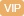| APP | 校园号 | 客服 客服热线：400-863-9889

400-863-9889（1）25×（8×3）
=25×8+25×3
=200+75
=275

25×（8×3）
=25×8×3
=200×3
=600
25×（8×3）
=25×8×3
=200×3
=600

（2）（40+12）×5
=40+12×5
=40+60
=100

（40+12）×5
=40×5+12×5
=200+60
=260
（40+12）×5
=40×5+12×5
=200+60
=260
【考点】整数四则混合运算【专题】运算顺序及法则．
【分析】判两种相关的量之间是否成正比就这两种量是否是对应的比一，如果是比一定，就成比例，如果是值一或比值不一定就不成比例．

【解答】解：设正方形的边长2，则正方内最大的圆的径是，

【点评】题属根据正、反比例的意义，识两种相关联的否成正比例，就看这两种量是否对的一定，再出断．

0/0